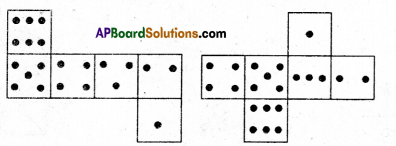# AP Board 7th Class Maths Solutions Chapter 14 Understanding 3D and 2D Shapes Ex 2

AP State Syllabus AP Board 7th Class Maths Solutions Chapter 14 Understanding 3D and 2D Shapes Ex 2 Textbook Questions and Answers.

## AP State Syllabus 7th Class Maths Solutions 14th Lesson Understanding 3D and 2D Shapes Exercise 2Question 1.
Some nets are given below. Trace them and paste them on a thick paper. Try to make 3-D
shapes by suitably folding them and gluing together. Match the net with it’s 3-D shape.
Nets
3D shapes
(i)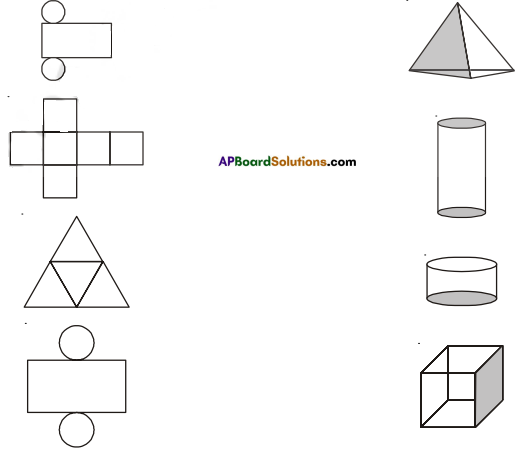Solution: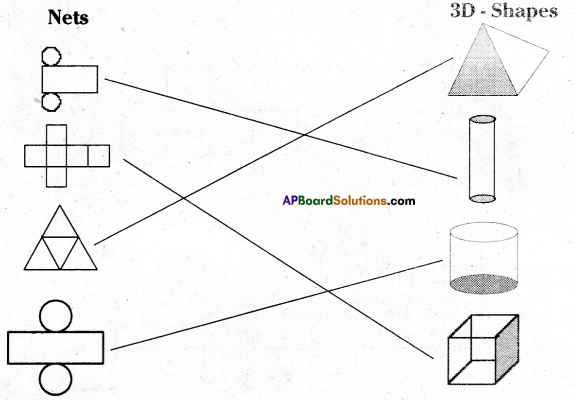Question 2.
Three nets for each shape are given here. Match the net with its 3-D shape.
Solution: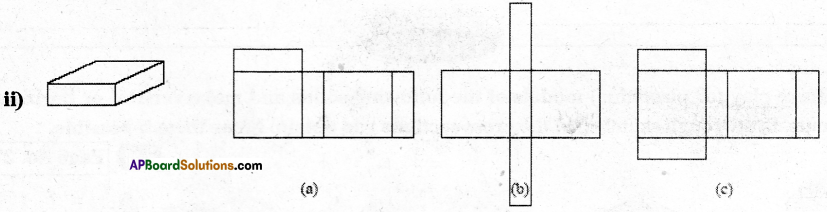Solution: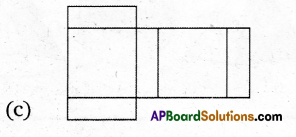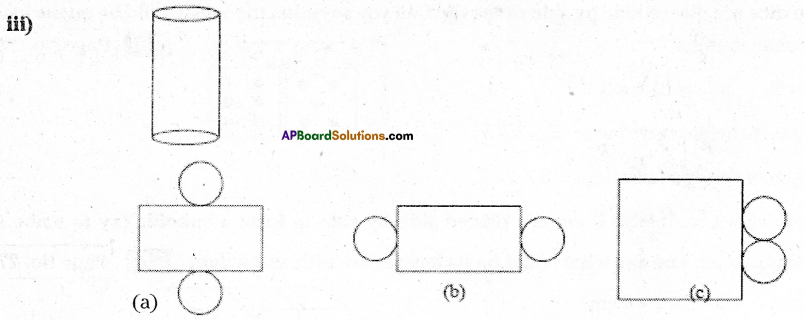Solution: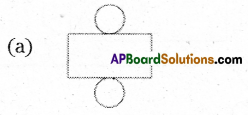Question 3.
A dice is a cube with dots on each face. The opposite faces of a dice always have a total of seven dots on them.
Here are two nets to make dice. Insert the suitable number of dots in blanks.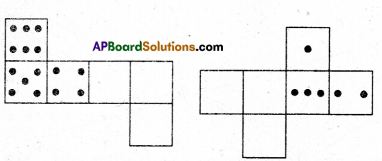Solution: